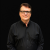## Saturday, October 6, 2012

### Lambda Calculus and Link Variables in the InfoSWMM, H2OMAP SWMM and SWMM 5 Dynamic Wave Solution

Subject:  Lambda Calculus and Link Variables in the InfoSWMM, H2OMAP SWMM and SWMM 5 Dynamic Wave Solution## Successive under-relaxation for the SWMM 5 Dynamic Wave Solution

Subject:  Successive under-relaxation for the SWMM 5 Dynamic Wave Solution
SWMM 5 uses the method of Successive under-relaxation to solve the Node Continuity Equation and the Link Momentum/Continuity Equation for a time step.  The dynamic wave solution in dynwave.c will use up to 8 iterations to reach convergence before moving onto the next time step.  The differences between the link flows and node depths are typically small (in a non pumping system) and normally converge within a few iterations unless you are using too large a time step.  The number of iterations is a minimum of two with the 1st iteration NOT using the under-relaxation parameter omega. The solution method can be term successive approximation, fixed iteration or Picard Iteration, fixed-point combinatory, iterated function and Lambda Calculus. In computer science, iterated functions occur as a special case of recursive functions, which in turn anchor the study of such broad topics as lambda calculus, or narrower ones, such as the denotational semantics
In the SWMM 5 application of this various named iteration process there are three main concepts for starting, iterating and stopping the iteration process during one time step:
·         The 1st guess of the new node depth or link flow is the current link flow (Figure 3) and the new estimated node depths and link flows are used at each iteration to estimate the new time step depth or flow.  For example, in the node depth (H) equation dH/dt = dQ/A the value of dQ or the change in flow and the value of A or Area is updated at each iteration based on the last iteration's value of all node depths and link flows.
·         A bound or a bracket on each node depth or link flow iteration value is used by averaging the last iteration value with the new iteration value.  This places a boundary on how fast a node depth or link flow can change per iteration – it is always ½ of the change during the iteration (Figure 1).

·         The Stopping Tolerance (Figure 2) determines how many iterations it takes to reach convergence and move out of the iteration process for this time step to the next time step.
Figure 1.  Under relaxation with an omega value of ½ is done on iterations 2 through a possible 8 in SWMM 5. This is not done for iteration 1.
Figure 2.  if the change in the Node Depth is less than the stopping tolerance in SWMM 5 the node is considered converged.  The stopping tolerance has a default value of 0.005 feet in SWMM 5.0.022.

Figure 3.  The differences between the link flows and node depths are typically small (in a non pumping system) and normally converge within a few iterations unless you are using too large a time step.  The number of iterations is a minimum of two with the 1stiteration NOT using the under-relaxation parameter omega.

St. Venant equation – this is the link attribute data used when the St. Venant Equation is used inSWMM 5, H2OMAP SWMM and InfoSWMM.  Simulated Parameters from the upstream, midpoint and downstream sections of the link are used.

Normal Flow Equation – this is the link attribute data used when the Normal Flow Equation is used in H2OMAP SWMM. Only simulated parameters from the upstream end of the link are used if the normal flow equation is used for the time step.  The normal flow equation is used if the flow is supercritical or the water surface slope is less than the bed slope of the link.

Non Linear Term in the Saint Venant Equation of SWMM 5

The flow equation has six components that have to be in balance at each time step:
1. The unsteady flow term or dQ/dt
2. The friction loss term (normally based on Manning's equation except for full force mains),
3. The bed slope term or dz/dx
4. The water surface slope term or dy/dx,
5. The non linear term or d(Q^2/A)/dx and
6. The entrance, exit and other loss terms.
All of these terms have to add up to zero at each time step. If the water surface slope becomes zero or negative then the only way the equation can be balanced is for the flow to decrease. If the spike is due to a change in the downstream head versus the upstream head then typically you will a dip in the flow graph as the water surface slope term becomes flat or negative, followed by a rise in the flow as the upstream head increases versus the downstream head.